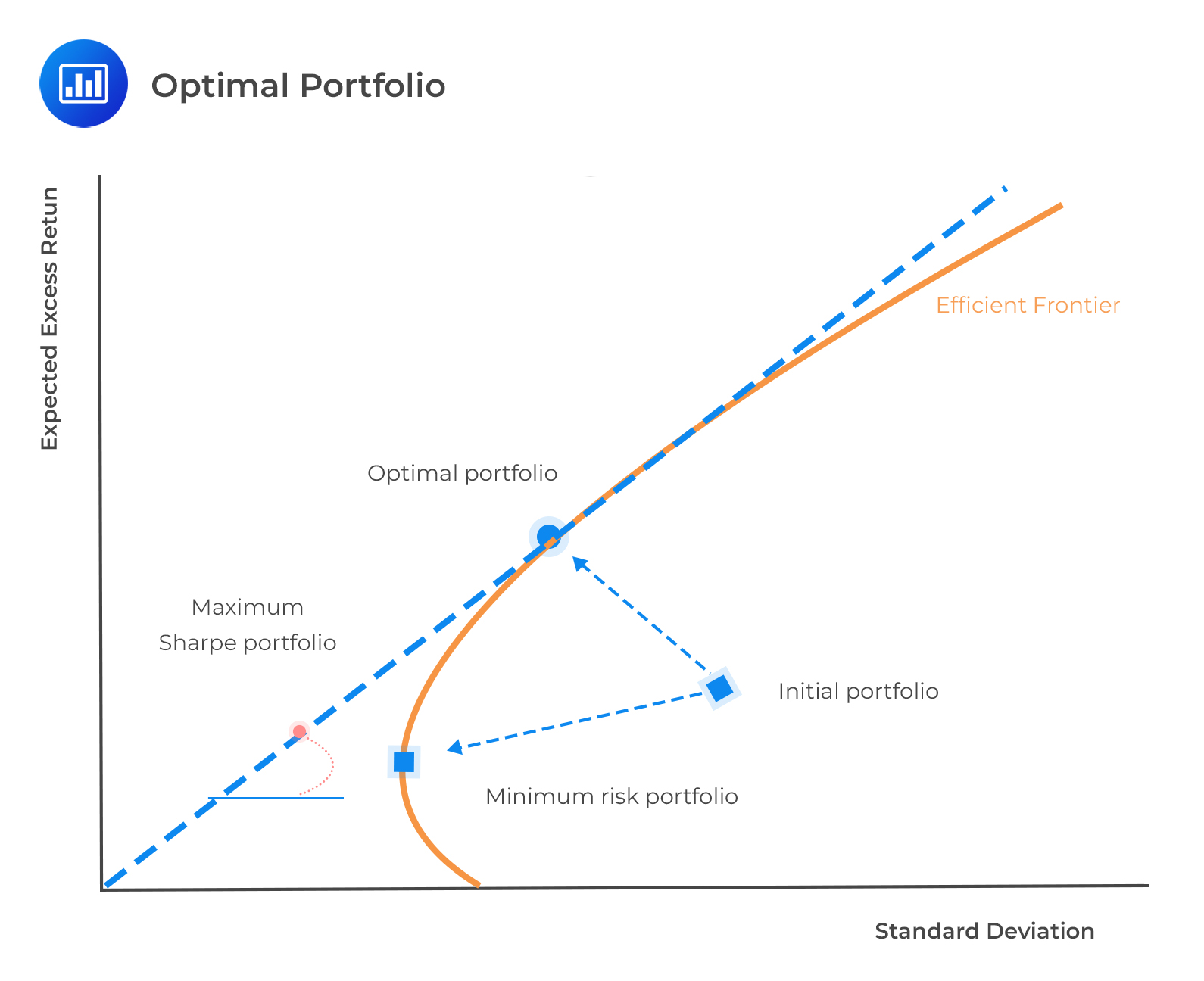# Application of the Information Ratio

An optimal portfolio can be obtained by combining a risk-free asset with an optimally risky asset portfolio. The optimally risky asset portfolio is the point where the capital allocation line is tangent to the efficient frontier.The optimal portfolio is the one with the highest possible Sharpe ratio. Therefore, irrespective of an investor’s risk aversion, they need to find a single risky asset portfolio with the highest Sharpe ratio, such that the following equation holds.

$$SR_P^2=SR_B^2+IR^2$$

Additionally, the portfolio with the highest information ratio has the highest Sharpe ratio. However, this may not be the ideal case when IR is negative. Consequently, the mean-variance theory asserts that the best criterion for active performance assessment is using the expected information ratio, especially when using the same benchmark.

The information ratio is also applied in the determination of the optimal level of active risk for unconstrained portfolios:

$$STD\left(R_A\right)=\frac{IR}{SR_B}{STD}(R_B)$$

#### Example: Optimal Level of Active Risk

Calculate the optimal level of aggressiveness in an actively managed portfolio with an information ratio of 0.20. Further, assume that the benchmark portfolio has a Sharpe ratio of 0.25 and a total risk of 15.0%

#### Solution

Using the equation:

\begin{align*} STD\left(R_A\right) & =\frac{IR}{SR_B}STD(R_B) \\ STD\left(R_A\right) & =\left(\frac{0.20}{0.25}\right)\times15\%=12.0\%. \\ \end{align*}

## Question

A portfolio manager uses a benchmark portfolio that has a Sharpe ratio of 0.30 and a total risk of 12.0%. His actively managed portfolio has an information ratio of 0.25 and an active risk of 5.0%. The portfolio’s optimal level of active risk is closest to:

1. 10%.
2. 12%.
3. 15%.

#### Solution

\begin{align*} STD\left(R_A\right) & =\frac{IR}{SR_B}STD(R_B) \\ STD\left(R_A\right) &=\left(\frac{0.25}{0.30}\right)12.0\%=10.0\%. \end{align*}

Reading 45: Analysis of Active Portfolio Management

LOS 45 (d) Explain how the information ratio may be useful in investment manager selection and choosing the level of active portfolio risk.

Shop CFA® Exam Prep

Offered by AnalystPrepLevel I
Level II
Level III
All Three Levels
Featured Shop FRM® Exam PrepFRM Part I
FRM Part II
FRM Part I & Part II
Learn with Us

Subscribe to our newsletter and keep up with the latest and greatest tips for success
Shop Actuarial Exams PrepExam P (Probability)
Exam FM (Financial Mathematics)
Exams P & FMGMAT® Complete CourseDaniel Glyn
2021-03-24
I have finished my FRM1 thanks to AnalystPrep. And now using AnalystPrep for my FRM2 preparation. Professor Forjan is brilliant. He gives such good explanations and analogies. And more than anything makes learning fun. A big thank you to Analystprep and Professor Forjan. 5 stars all the way!michael walshe
2021-03-18
Professor James' videos are excellent for understanding the underlying theories behind financial engineering / financial analysis. The AnalystPrep videos were better than any of the others that I searched through on YouTube for providing a clear explanation of some concepts, such as Portfolio theory, CAPM, and Arbitrage Pricing theory. Watching these cleared up many of the unclarities I had in my head. Highly recommended.Nyka Smith
2021-02-18
Every concept is very well explained by Nilay Arun. kudos to you man!2021-02-13